# Introduction to Trigonometry

chapter 8. Introduction to Trigonometry .
1. The word ‘trigonometry’ is derived from the Greek words ‘tri’ (meaning three), ‘gon’ (meaning sides) and ‘metron’ (meaning measure). It is the study of relationships between the sides and angles of a triangle.

2. Early astronomers used it to find out the distances of the stars and planets from the Earth.Today, most of the technologically advanced methods used in Engineering and Physical Sciences are based on trigonometrical concepts.

Steps to find the trigonometric ratios with respect to an angle (say A) in a right angled triangle:

a. Keep triangle in such a way so that angle A is in the base (along with the 90 degree angle).
b. Identify the “perpendicular” (p), “base” (b) and hypotenuse (h) sides. Usually two of the sides are given; the unknown can be calculated using Pythagoras Theorem.
c. Find out the required trigonometric ratio. (Refer to the video to find out the shortcut to remember the six trigonometric ratios.)

# Introduction To Trigonometry – Class X – Part 1 | Exercise 8.1 Q1-4 Solved

1. In Δ ABC, right-angled at B, AB = 24 cm, BC = 7 cm. Determine :

(i) sin A, cos A
(ii) sin C, cos C

2. In Fig. 8.13, find tan P – cot R. (For figure refer to the question in video).

3. If sin A = 3/4 , calculate cos A and tan A.

4. Given 15 cot A = 8, find sin A and sec A.

# Introduction To Trigonometry – Class X – Part 2 | Exercise 8.1 Q5-9 Solved

5. Given sec θ =13 / 12, calculate all other trigonometric ratios.

6. If ∠ A and ∠ B are acute angles such that cos A = cos B, then show that ∠ A = ∠ B.

7. If cot θ =7 / 8 .
Evaluate :

(i)(1+ sinθ) (1 – sinθ )/(1+ cosθ) (1 – cosθ )
(ii) (cotθ)(cotθ) [Read as cot square theta]

8. If 3 cot A = 4, check whether [1-(tanA)^2]/[1+(tanA)^2] = (cosA)^2 – (sinA)^2

9. In triangle ABC, right-angled at B, if tan A =1/(root3), find the value of

(i) sin A cos C + cos A sin C
(ii) cos A cos C – sin A sin C

# Introduction To Trigonometry – Class X – Part 3 | Exercise 8.1 Q10-11 Solved

10. In Δ PQR, right-angled at Q, PR + QR = 25 cm and PQ = 5 cm. Determine the values of sin P, cos P and tan P.

11. State whether the following are true or false. Justify your answer.

(i) The value of tan A is always less than 1.
(ii) sec A =12/5 for some value of angle A.
(iii) cos A is the abbreviation used for the cosecant of angle A.
(iv) cot A is the product of cot and A.
(v) sin θ =4/3 for some angle θ.

How to remember trigonometric ratios for specific angles: 0°, 30°, 45°, 60°, 90°:

a) Just remember the values for Sin of the angles.
b) The values for Cos will be in reverse order of Sin of the angles.
c) Tan of angle = Sin of angle/ Cos of angle
d) Cosec is reciprocal of Sin.
e) Sec is reciprocal of cos.
f) Cot is reciprocal of tan.

# Introduction To Trigonometry – Class X – Part 4 | Exercise 8.2 Q1 Solved

1. Evaluate the following :

(i) sin 60° cos 30° + sin 30° cos 60°

(ii) 2 tan2 45° + cos2 30° – sin2 60°

(iii) cos 45° / (sec 30° + cosec 30°)

(iv) (sin 30° + tan 45° – cosec 60°) / (sec 30° + cos 60° + cot 45°)

(v) (5 cos2 60° + 4 sec2 30° – tan2 45°)/ (sin2 30° + cos2 30°)

# Introduction to Trigonometry – Class X – Part 5 | Exercise 8.2 Q2-4 Solved

2. Choose the correct option and justify your choice :

(i) 2 tan 30°/ (1 + tan2 30°) =

(A) sin 60° (B) cos 60° (C) tan 60° (D) sin 30°

(ii) (1 – tan2 45°)/ (1 + tan2 45°) =

(A) tan 90° (B) 1 (C) sin 45° (D) 0

(iii) sin 2A = 2 sin A is true when A =

(A) 0° (B) 30° (C) 45° (D) 60°

(iv) 2 tan 30°/ (1 – tan2 30°) =

(A) cos 60° (B) sin 60° (C) tan 60° (D) sin 30°

3. If tan (A + B) = root3 and tan (A – B) = 1/root3 ; 0° ＜ A + B ＜=90°; A ＞ B, find A and B.

4. State whether the following are true or false. Justify your answer.

(i) sin (A + B) = sin A + sin B.

(ii) The value of sin θ increases as θ increases.

(iii) The value of cos θ increases as θ increases.

(iv) sin θ = cos θ for all values of θ.

(v) cot A is not defined for A = 0°.

How to solve trigonometric ratios for complementary angles?

a) Identify the complementary angles by adding (any) two angles – it is always a pair of angles that are complementary to each other.

b) Convert one of the trigonometric ratio to another

— If the question statement consists of a mixture of sin and cos, convert sin into cos (or the other way round) and evaluate further.
— If the question statement consists of a mixture of tan and cot, convert cot into tan (or the other way round) and evaluate further. Product of tan and cot of the same angle is always one.
— If the question statement consists of a mixture of cosec and sec, convert sec into cosec (or the other way round) and evaluate further.

c) Please remember that the trigonometric ratios for specific angles: 0°,30°,45°, 60°, 90° are generally not involved as then finding the value would be simple. The questions usually involve different involves – for example : angles 18 and 72 or 24 and 66.

# Introduction to Trigonometry – Class X – Part 6 | Exercise 8.3 Q1-7 Solved

1. Evaluate

(i) sin 18°/ cos 72°

(i) tan 26°/ cot 64°

(iii) cos 48° – sin 42°

(iv) cosec 31° – sec 59°

2. Show that :

(i) tan 48° tan 23° tan 42° tan 67° = 1

(ii) cos 38° cos 52° – sin 38° sin 52° = 0

3. If tan 2A = cot (A – 18°), where 2A is an acute angle, find the value of A.

4. If tan A = cot B, prove that A + B = 90°.

5. If sec 4A = cosec (A – 20°), where 4A is an acute angle, find the value of A.

6. If A, B and C are interior angles of a triangle ABC, then show that Sin (B+C)/2 = Cos A/2

7. Express sin 67° + cos 75° in terms of trigonometric ratios of angles between 0° and 45°.

1. Trigonometric identities that are in syllabus (class 10, NCERT):

sin²θ + cos²θ = 1

1 + cot²θ = cosec²θ

1 + tan²θ = sec²θ

2. How to remember trigonometric identities: As explained in the video, just remember the first one i.e sin²θ + cos²θ = 1

a) The second one (1 + cot²θ = cosec²θ) can be derived from the first by dividing both sides by sin²θ.

b) The second one (1 + tan²θ = sec²θ) can be derived from the first by dividing both sides by cos²θ.

3. Usually the concepts of “trigonometric angles for complementary angles” and “trigonometric identities” are tested together in exam questions.

# Introduction to Trigonometry – Class X – Part 7 | Exercise 8.4 Q1-3 Solved

1. Express the trigonometric ratios sin A, sec A and tan A in terms of cot A.

2. Write all the other trigonometric ratios of ∠ A in terms of sec A.

3. Evaluate :

(i) (sin²63° + sin²27°) / (cos²17° + cos²73°)

(ii) sin 25°cos 65° + cos 25°sin 65°

# Introduction to Trigonometry – Class X – Part 8 | Exercise 8.4 Q4- 5 (iii) Solved

4. (i) 9 sec²A – 9 tan²A =

(A) 1                      (B) 9                    (C) 8                      (D) 0

(ii) (1 + tanθ + secθ) (1 + cotθ – cosecθ) =

(A) 0                        (B) 1                    (C) 2                     (D) –1

(iii) (sec A + tan A) (1 – sin A) =

(A) sec A                (B) sin A              (C) cosec A           (D) cos A

(iv) (1 + tan²A)/(1 + cot²A)

(A) sec²A               (B) –1                   (C) cot²A             (D) tan²A

5. Prove the following identities, where the angles involved are acute angles for which the expressions are defined.

(i) (cosec θ – cot θ)² = (1 – cosθ)/(1 + cosθ)

(ii) cosA /(1 + sinA) + (1 + sinA)/cosA = 2 secA

(iii) tanθ/(1 – cot θ) + cotθ/(1 – tanθ) = 1 + secθcosecθ

# Introduction to Trigonometry – Class X – Part 9 | Exercise 8.4 Q5 (iv) – 5 (x) Solved

(iv)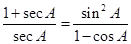(v)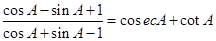, using the identity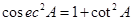(vi)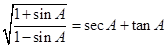(vii)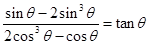(viii)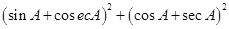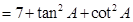(ix)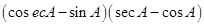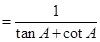(x)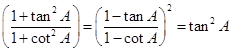More questions on Trigonometry: